## RS Aggarwal Class 6 Solutions Chapter 7 Decimals Ex 7C

These Solutions are part of RS Aggarwal Solutions Class 6. Here we have given RS Aggarwal Solutions Class 6 Chapter 7 Decimals Ex 7C.

Other Exercises

Question 1.
Solution:
9.6, 14.8, 37 and 5.9
Converting these decimals into like decimals and then adding 9.6 + 14.8 + 37.0 + 5.9
= 67.3 Ans.
Working :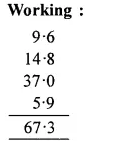Question 2.
Solution:
23.7, 106.94, 68.9 and 29.5
Converting them into like decimals and then adding
23.70 + 106.94 + 68.90 + 29.50
= 229.04 Ans.
Working :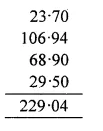Question 3.
Solution:
72.8, 7.68, 16.23 and 0.7
Converting them into like decimals and then adding
72.80 + 7.68 + 16.23 + 0.70
= 97.41 Ans.
Working :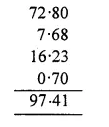Question 4.
Solution:
18.6, 84.75, 8.345 and 9.7
Converting them into like decimals and then adding
18.600 + 84.750 + 8.345 + 9.700
= 121.395 Ans.
Working :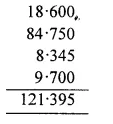Question 5.
Solution:
8.236, 16.064, 63.8 and 27.53
Converting them into like decimals and then adding
8.236 + 16.064 + 63.800 + 27.530
= 115.630 Ans.
Working :Question 6.
Solution:
28.9, 19.64, 123.697 and 0.354
Converting them into like decimals and then adding
28.900 + 19.640 + 123.697 + 0.354
= 172.591 Ans.
Working :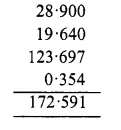Question 7.
Solution:
4.37, 9.638, 17.007 and 6.8
Converting them into like decimals and then adding
4. 370 + 9.638 + 17.007 + 6.800
= 37.815 Ans.
Working :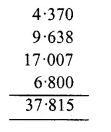Question 8.
Solution:
14.5, 0.038, 118.573 and 6.84
Converting them into like decimals and then adding
14.500 + 0.038 + 118.573 + 6.840
= 139.951 Ans.
Working :Question 9.
Solution:
Earning for the first day = 32.60 rupees
Earning for the second day = 56.80 rupees
Earning for the third day = 72 rupees
Total earning = Rs. 32.60 + Rs. 56.80 + Rs. 72
= Rs. 161.40 Ans.
WorkingQuestion 10.
Solution:
Cost of almirah = Rs. 11025
Cartage = Rs. 172.50
Cost on repair = Rs. 64.80
Total cost = Rs. 11025 + Rs. 172.50 + Rs. 64.80
= Rs. 11262.30 Ans.
Working :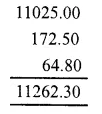Question 11.
Solution:
Distance covered by taxi = 36 km 235 m
= 36.235 km
Distance covered by Rickshaw = 4 km 85 m
= 4.085 km
and distance covered on foot
= 1 km 80 m
= 1.080 m
Total distance covered = 36.235 km + 4.085 km + 1.080 km
= 41.400 km
= 41 km 400 m Ans.
Working :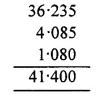Question 12.
Solution:
Weight of sugar in a bag = 45 kg 80 g
= 45.080 kg
Mass (weight) of empty bag = 950 g
= 0.950 kg
Total weight of the bag with sugar = 45 kg 80 g + 950 g
= 45.080 kg + 0.950 kg
= 46.030 kg
= 46 kg 30 g Ans.
Working :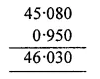Question 13.
Solution:
Length of cloth for shirt = 2 m 70 cm
= 2.70 m
Length of cloth for pyjamas = 2 m 60 cm
= 2.60 m
Total length of cloth = 2.70 m + 2.60 m
= 5.30 m
= 5 m 30 cm Ans.
Working :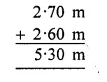Question 14.
Solution:
Cloth of salwar = 2 m 5 cm = 2.05 m
Cloth for shirt = 3 m 35 cm = 3.35 m
Total length of cloth = 2.05 m + 3.35 m
= 5.4.0 m
= 5 m 40 cm Ans.
Working :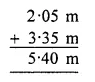Hope given RS Aggarwal Solutions Class 6 Chapter 7 Decimals Ex 7C are helpful to complete your math homework.

If you have any doubts, please comment below. Learn Insta try to provide online math tutoring for you.